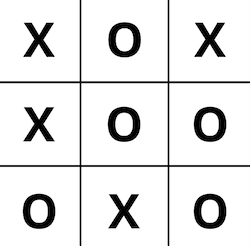# Multidimensional Arrays in Elixir

## April 23, 2016

I recently picked up a copy of Game Programming Patterns, and started messing around with implementing some of them in Elixir. I very quickly ran into some trouble dealing with multidimensional arrays.

Take the game of Tic-tac-toe for example.Most programming languages would represent this Tic-tac-toe board as a multidimensional array, like this:

``````var board = [
["x", "o", "x"],
["x", "o", "o"],
["o", "x", "o"]
]
``````

You could then access and change squares on the board with the `board[x][y]` syntax.

``````board // "x"
board = "o"
``````

However, if you try this in Elixir, it won’t work.

``````board = [
["x", "o", "x"],
["x", "o", "o"],
["o", "x", "o"]
]

board
** (ArgumentError) the Access calls for keywords expect the key to be an atom, got: 0
(elixir) lib/access.ex:136: Access.fetch/2
(elixir) lib/access.ex:149: Access.get/3
i

board = "y"
** (CompileError) iex:3: cannot invoke remote function Access.get/2 inside match
(stdlib) lists.erl:1353: :lists.mapfoldl/3
``````

This is for several reasons. First of all, Elixir’s `[]` syntax is powered by the Access behaviour, which is only implemented for maps and keyword lists. So, if you wanted to get the value of the game board at coords `[0,0]`, while representing the board as an ordinary list, you’d need to do this:

``````board
|> Enum.at(0)
|> Enum.at(0)
# => "x"
``````

It’s no easier if you decide to use a multidimensional tuple to represent the board; you just have to use the `elem/2` function instead of `Enum.at/2`:

``````board = {
{"x", "o", "x"},
{"x", "o", "o"},
{"o", "x", "o"}
}

board
|> elem(0)
|> elem(0)
# => "x"
``````

While this is a little weird, it’s manageable for reading from the board. But what about writing to the board? That’s where the second difficulty comes in.

Elixir variables are immutable. This means that we can’t just mutate the game board variable at the `[0,0]` coords, we have to make a modified copy of the entire board.

This is easy to do in a one-dimensonal list or tuple:

``````# Use List.replace_at/3 for lists
modified = List.replace_at(list, 0, "y")

# And put_elem/3 for tuples
modified = put_elem(tuple, 0, "y")
``````

However, it becomes pretty complicated when you need to write to a position in a nested list. In that case, you would need to:

1. Get the nested list you need to modify out of the container list.
2. Make a modified copy of the nested list.
3. Recursively rebuild the entire data structure.

Every nested Elixir data structure has this problem, and the good news is that Elixir has macros that do all of this for you. See the `put_in` macro, for example:

``````map = %{user: %{age: 20}}
put_in map[:user][:age], 21
# => %{user: %{age: 21}}
``````

The bad news is that the `put_in` macro also relies on the Access behaviour, and therefore only works on maps and keyword lists, not regular lists and tuples. What to do?

## Solution: Use a Map

We can eliminate all of these difficulties if we represent the game board as a map rather than a list or tuple.

``````board = %{
0 => %{0 => "x", 1 => "o", 2 => "x"},
1 => %{0 => "x", 1 => "o", 2 => "o"},
2 => %{0 => "o", 1 => "x", 2 => "o"}
}
``````

The keys of each nested map are zero-indexed, just like arrays in other languages. This allows you to use the Access behaviour:

``````board # => "x"
``````

It also allows you to use the `put_in` macro.

``````board = put_in board, "y"
``````

This is just about as user-friendly as other languages, but it is still harder to manually write this map structure than it would be to write a nested list.

We can make the experience nicer by creating a module that can generate these maps from lists and vice versa.

``````defmodule Matrix do
@moduledoc """
Helpers for working with multidimensional lists, also called matrices.
"""

@doc """
Converts a multidimensional list into a zero-indexed map.

## Example

iex> list = [["x", "o", "x"]]
...> Matrix.from_list(list)
%{0 => %{0 => "x", 1 => "o", 2 => "x"}}
"""
def from_list(list) when is_list(list) do
do_from_list(list)
end

defp do_from_list(list, map \\ %{}, index \\ 0)
defp do_from_list([], map, _index), do: map
defp do_from_list([h|t], map, index) do
map = Map.put(map, index, do_from_list(h))
do_from_list(t, map, index + 1)
end
defp do_from_list(other, _, _), do: other

@doc """
Converts a zero-indexed map into a multidimensional list.

## Example

iex> matrix = %{0 => %{0 => "x", 1 => "o", 2 => "x"}}
...> Matrix.to_list(matrix)
[["x", "o", "x"]]
"""
def to_list(matrix) when is_map(matrix) do
do_to_list(matrix)
end

defp do_to_list(matrix) when is_map(matrix) do
for {_index, value} <- matrix,
into: [],
do: do_to_list(value)
end
defp do_to_list(other), do: other
end
``````

## Conclusion

With the `Matrix` module in place, we can now manipulate multidimensional arrays just as well as other languages: 1

``````# Create the game board
board = Matrix.from_list([
["x", "o", "x"],
["x", "o", "o"],
["o", "x", "o"]
])

# Access a value with coords
board # => "o"

# Update the value at coords
board = put_in board, "x"

# Get a multidimensional list back:
Matrix.to_list(board)
# [
#   ["x", "o", "x"],
#   ["x", "o", "o"],
#   ["x", "x", "o"]
# ]
``````

It would be nice if something like this was included in the standard library, but perhaps it isn’t a common enough problem. Anyway, I hope it was helpful!

1. This approach will still consume more memory to update the game board than other languages would because we copy the board for each mutation, rather than mutate the board in place. You can fix this by representing each square on the board with an Agent, but this slows down reading the state of the board. It’s a tradeoff. [return]
comments powered by Disqus